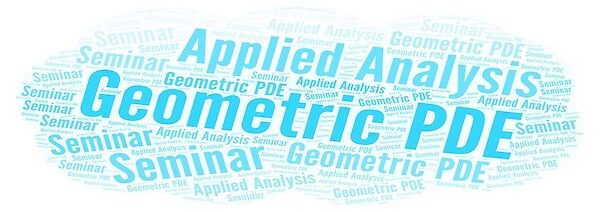# [Seminar] "Several topics on the linear transport equation" by Prof. Kohei Soga### Date

2023年9月7日 (木) 16:00 17:00

C209

### Description

Geometric PDE and Applied Analysis Seminar (September 7, 2023)

Title: Several topics on the linear transport equation

Speaker: Prof. Kohei Soga (Keio University)

Abstract: The linear transport equation (LTE), arising from fluid dynamics, governs evolution of the density of incompressible fluid or evolution of the level-set function that gives a material interface of two immiscible fluids, where the coefficient in LTE is the velocity field derived from Navier-Stokes (type) equations. If regularity of the velocity field is Sobolev, which is quite natural in terms of analysis of Navier-Stokes equations, LTE turns out to be non-trivial; even if the velocity field is smooth, LTE in computational fluid dynamics exhibits some difficulty in regards to numerical calculation of geometric quantities of the level-set. In this talk, I will speak about roles of LTE in fluid dynamics and mathematical analysis on
(1) Finite difference methods for DiPerna-Lions weak solutions to LTE with Sobolev velocity fields;
(2) Global weak solutions to LTE-Navier-Stokes type model with mass diffusion;
(3) Nonlinear modifications of LTE as the level-set equation and their solvability (classical & viscosity solutions).

All-OIST Category: